## S4B1 - Graduate Seminar- The ubiquity of gaussian measures

Prof. M. Disertori, Susanne Hilger

Time/location: Wednesday 12-14  in room N0.008

The first seminar will take place on Wednesday April 10

Description. Perturbations of Gaussian measures arise in a wide range of fields of mathematics and theoretical physics. In the context of statistical mechanics and theoretical physics, (discrete) Gaussian measures often take the form

dμC (φ) = dφ e−(φ,C-1 φ)

where φ : Λ → H, with Λ ⊂ Zd , H is a real or complex finite dimensional
Hilbert space, C : Λ × Λ → C is an invertible matrix and (φ, C−1 φ) = ∑ij∈Λ Cij (φi , φj )H .
In this seminar we plan to learn how these measures are related with
some of the most famous models in statistical mechanics.

We will start with a selection of topics from Chapters 8 and 9 of the
book Statistical Mechanics of Lattice Systems: a Concrete Mathematical
Introduction S. Friedli and Y. Velenik (available online here). Chapter 8 deals
with Gaussian free fields, and explains how Gaussian measures can be used to
describe random walks on the lattice. Chapter 9 presents the so called O(n)
models (the prominent examples being Ising, XY and Heisenberg model).
For n > 1, these models are at the core of most theories with continuous
symmetry both in statistical mechanics and field theory. The main result of
this chapter is the celebrated Mermin-Wagner theorem.

According to time/interest we may then read parts of the lecture notes
by D. Brydges Self-Interacting Walk and Functional Integration, relating
random walks with interaction to a generalized version of the Gaussian in-
tegral.

Possible additional (alternative) topics include: duality transformations
mapping nonGaussian to Gaussian models, and Gaussian measures on ma-
trices.

Prerequisites. Functional analysis. Some basic knowledge in probability
may be useful but is not necessary. The necessary preliminary notions on
random walks and probability are all summarized Appendix B.8 B.9 and
B.13 of the book by Friedli and Velenik.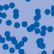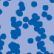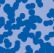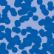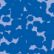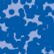## News

Prof. Dr. Andreas Eberle erhält den diesjährigen Lehrpreis der Universität Bonn. (22.07.2020)

Herr Dr. Richard Höfer erhielt den Hausdorff-Gedächtnispreis 2019 der Fachgruppe Mathematik für die beste Disseration. Betreut wurde die Arbeit von Prof. J. Velázquez (29.01.2020).

Einer der Preise der Bonner Mathematischen Gesellschaft (BMG) für die besten Bachelorarbeiten 2019 ging an Herrn Peter Holderrieth unter der Betreuung von Prof. A. Eberle (29.01.2020).

Frau Dr. Beate Doerffel hat einen Lehrpreis der Mathematisch-Naturwissenschaftlichen Fakultät 2019 erhalten (05.12.2019).

Contact

Managing Director: Prof. Dr. Massimiliano Gubinelli